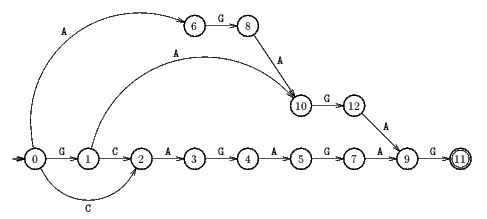Next: BNDM algorithm Up: ESMAJ Prev: Turbo Reverse Factor algorithm

# Forward Dawg Matching algorithm

Main features
• uses the suffix automaton of x;
• O(n) worst case time complexity;
• performs exactly n text character inspections.
Description

The Forward Dawg Matching algorithm computes the longest factor of the pattern ending at each position in the text. This is make possible by the use of the smallest suffix automaton (also called DAWG for Directed Acyclic Word Graph) of the pattern. The smallest suffix automaton of a word w is a Deterministic Finite Automaton S(w) = (Q, q0, T, E). The language accepted by S(w) is L(S(w))={u in* :  exists v in* such that w=vu}.

The preprocessing phase of the Forward Dawg Matching algorithm consists in computing the smallest suffix automaton for the pattern x. It is linear in time and space in the length of the pattern.

During the searching phase the Forward Dawg Matching algorithm parses the characters of the text from left to right with the automaton S(x) starting with state q0. For each state q in S(x) the longest path from q0 to p is denoted by length(q). This structure extensively uses the notion of suffix links. For each state p the suffix link of p is denoted by S[p]. For a state p, let Path(p)=(p0,p1, ... ,p) be the suffix path of p such that p0=p, for 1i, pi=S[pi-1] and p=q0. For each text character y[j] sequentially, let p be the current state, then the Forward Dawg Matching algorithm takes a transition defined for y[j] for the first state of Path(p) for which such a transition is defined. The current state p is updated with the target state of this transition or with the initial state q0 if no transition exists labelled with y[j] from a state of Path(p).

An occurrence of x is found when length(p)=m.

The Forward Dawg Matching algorithm performs exactly n text character inspections.

The C code

The function buildSuffixAutomaton is given chapter Reverse Factor algorithm. All the other functions to build and manipulate the suffix automaton can be found in the introduction, section implementation.

```int FDM(char *x, int m, char *y, int n) {
int j, init, ell, state;
Graph aut;

/* Preprocessing */
aut = newSuffixAutomaton(2*(m + 2), 2*(m + 2)*ASIZE);
buildSuffixAutomaton(x, m, aut);
init = getInitial(aut);

/* Searching */
ell = 0;
state = init;
for (j = 0; j < n; ++j) {
if (getTarget(aut, state, y[j]) != UNDEFINED) {
++ell;
state = getTarget(aut, state, y[j]);
}
else {
while (state != init &&
getTarget(aut, state, y[j]) == UNDEFINED)
if (getTarget(aut, state, y[j]) != UNDEFINED) {
ell = getLength(aut, state) + 1;
state = getTarget(aut, state, y[j]);
}
else {
ell = 0;
state = init;
}
}
if (ell == m)
OUTPUT(j - m + 1);
}
}

```
The example

Preprocessing phaseSuffix automaton used by Forward Dawg Matching Search algorithm.

Searching phase

References
• CROCHEMORE, M., RYTTER, W., 1994, Text Algorithms, Oxford University Press.Next: BNDM algorithm Up: ESMAJ Prev: Turbo Reverse Factor algorithm

e-mails: {Christian.Charras, Thierry.Lecroq }@laposte.net
Tue Jan 14 15:03:31 MET 1997